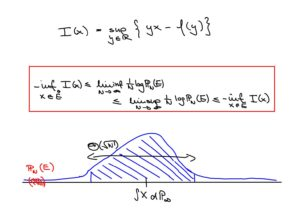# Dr. Christian Mönch — Research

## Research areas

### - Complex networks

• scale-free networks
• spatially extendend networks
• directed random graphs
• stochastic processes on (random) graphs

For an introduction to/ overview of this area, see this course taught in winter 2019/20 and the associated webpage.### - Persistence probabilities

This area of probability theory is concerned with problems of the following type: Given a real valued stochastic process whose range almost surely contains (a dense subset of) the whole real line, how rare is the event that it stays, say, below a given threshold for a certain period of time? For Markov processes the answer is given by classical fluctuation theory, but for non-Markovian processes the picture is very far from completion.For an introduction / survey of this field see this article by F. Aurzada & T. Simon.

### - Large deviations

Large deviation theory is a unified framework to study the probabilities of rare events in stochastic systems. Its development was initiated by S.R.S. Varadhan in the 1960s. See these lecture notes by W. König for an introduction.## Publications & preprints

1. Stein Andreas Bethuelsen, Christian Hirsch and CM. Invariance principle for random walks on dynamically averaging random conductances. Preprint, 2020.
2. Peter Gracar, Markus Heydenreich, CM and Peter Mörters. Transience Versus Recurrence for Scale-Free Spatial Networks. Algorithms and Models for the Web Graph. WAW 2020. Lecture Notes in Computer Science, vol 12091, 2020
3. Peter Gracar, Markus Heydenreich, CM and Peter Mörters. Recurrence versus transience for Weight-dependent Random Connection Models. Preprint, 2019.
4. CM. Self-similar co-ascent processes and Palm calculus. Preprint, 2019.
5. CM. Universality for persistence exponents of local times of self-similar processes with stationary increments. Preprint, 2018.
6. Christian Hirsch and CM. Distances and large deviations in the spatial preferential attachment model. Bernoulli 26 (2020), no. 2, 927--947. MR4058356
7. Frank Aurzada and CM. Persistence probabilities and a decorrelation inequality for the Rosenblatt process and Hermite processes. Теория вероятностей и ее применения 63 (2018), pp. 817-826 and Theory of Probability and its Applications 63.4 (2019), pp. 664-670. MR3869634
8. Steffen Dereich, CM and Peter Mörters. Distances in scale-free networks at criticality. Electronic Journal of Probability 22 (2017), paper no. 77, 38 pp. MR3710797
9. CM. Conditionally Poissonian random digraphs. Preprint, 2017.
10. CM. Persistence of activity in critical scale free Boolean networks. Tagungsbericht/ extended abstract, Oberwolfach Report 35 (2015), pp. 2020–2023.
11. Vaios Laschos and CM. Relations between L^p- and pointwise convergence of families of functions indexed by the unit interval. Real Analysis Exchange, 38.1 (2012/13) pp. 177–192. MR3083205
12. Steffen Dereich, CM and Peter Mörters. Typical distances in ultrasmall random networks. Advances in Applied Probability, 44.2 (2012), pp. 583–601. MR2977409

## Theses

• Distances in preferential attachment networks. PhD thesis, December 2013, supervised by Prof. Peter Mörters, University of Bath.
• Large deviations for the empirical pair measure of tree indexed Markov chains. Diploma thesis, April 2009, supervised by Prof. Heinrich von Weizsäcker, Technische Universität Kaiserslautern.

<< Back to main page